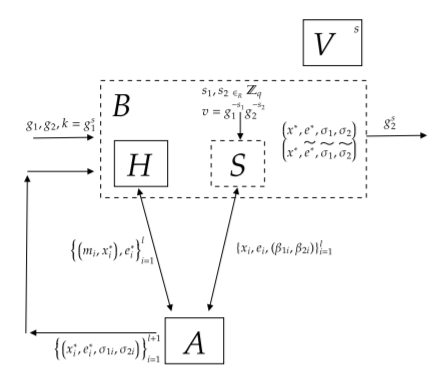Like   Tweet   Pin   +1   in
 /* styles */ Please feel free forward to your colleagues and share the news of our journal
 /* styles */ Dear Colleague, Welcome to our journal newsletter for Journal of Computer Security (JCS). We are delighted to announce the publication of Volume 29, Issue 2. The content from this new issue is included below, as well as details on how to submit your research to the journal. Looking forward to working with you.With kind regards,

Editors-in-Chief

Véronique Cortier
CNRS, Loria, France

Peng Liu
Pennsylvania State University, USA

 table div table+table+table+table+table div table{width:100%;padding:0}table div table+table+table+table+table div table img{width:96.23%;padding:0;float:none}table div table+table+table+table+table div table td{width:100%;padding:0 1.88% 18px}/* styles */# Volume 29, Issue 2

Research perspectives on fully homomorphic encryption models for cloud sector
Mittal, Sonam | Ramkumar, K.R.

A permission-dependent type system for secure information flow analysis – Openly Available
Xu, Zhiwu | Chen, Hongxu | Tiu, Alwen | Liu, Yang | Sareen, Kunal

Publicly auditable conditional blind signatures
Grontas, Panagiotis | Pagourtzis, Aris | Zacharakis, Alexandros | Zhang, Bingsheng

 table div table+table+table+table+table+table+table+table div table{width:100%;padding:0}table div table+table+table+table+table+table+table+table div table img{width:96.23%;padding:0;float:none}table div table+table+table+table+table+table+table+table div table td{width:100%;padding:0 1.88% 18px}/* styles */# Call for Papers

As a member of our research community, we would like to invite you to contribute your own work for publication in the journal. Journal of Computer Security offers contributing authors many benefits, including:

· First class Editorial Board

· Rigorous peer review and speedy manuscript processing

View the detailed Instructions to Authors.

 table div table+table+table+table+table+table+table+table+table+table+table div table{width:100%;padding:0}table div table+table+table+table+table+table+table+table+table+table+table div table img{width:96.23%;padding:0;float:none}table div table+table+table+table+table+table+table+table+table+table+table div table td{width:100%;padding:0 1.88% 18px}/* styles */# Related publications

 table div table+table+table+table+table+table+table+table+table+table+table+table+table div table{width:100%;padding:0}table div table+table+table+table+table+table+table+table+table+table+table+table+table div table table{padding:0;float:left!important;width:33.901%!important}table div table+table+table+table+table+table+table+table+table+table+table+table+table div table td{padding-left:30px;padding-right:30px}table div table+table+table+table+table+table+table+table+table+table+table+table+table div table table td,table div table+table+table+table+table+table+table+table+table+table+table+table+table div table table+table td{padding-left:0;padding-right:20px}table div table+table+table+table+table+table+table+table+table+table+table+table+table div table table+table{float:left!important;width:33.688%!important}table div table+table+table+table+table+table+table+table+table+table+table+table+table div table table+table+table{float:left!important;width:32.411%!important}table div table+table+table+table+table+table+table+table+table+table+table+table+table div table table+table+table td{padding-left:0;padding-right:0}/* styles */
 table div table+table+table+table+table+table+table+table+table+table+table+table+table+table+table div table{width:100%;padding:0}table div table+table+table+table+table+table+table+table+table+table+table+table+table+table+table div table img{width:96.23%;padding:0;float:none}table div table+table+table+table+table+table+table+table+table+table+table+table+table+table+table div table td{width:100%;padding:0 1.88% 18px}/* styles */table div table+table+table+table+table+table+table+table+table+table+table+table+table+table+table+table+table+table div table{width:100%;padding:0}table div table+table+table+table+table+table+table+table+table+table+table+table+table+table+table+table+table+table div table img{width:96.23%;padding:0;float:none}table div table+table+table+table+table+table+table+table+table+table+table+table+table+table+table+table+table+table div table td{width:100%;padding:0 1.88% 18px}/* styles */table div table+table+table+table+table+table+table+table+table+table+table+table+table+table+table+table+table+table+table+table+table+table+table div table{width:100%;padding:0}table div table+table+table+table+table+table+table+table+table+table+table+table+table+table+table+table+table+table+table+table+table+table+table div table img{width:96.23%;padding:0;float:none}table div table+table+table+table+table+table+table+table+table+table+table+table+table+table+table+table+table+table+table+table+table+table+table div table td{width:100%;padding:0 1.88% 18px}/* styles */